# Chapter 7 – Geometry

Consider the following statements and click to reveal the answer.

1. How many different quadrilaterals can you name which have at least the fol­lowing characteristics?

• Opposite sides equal in length and parallel
• Opposite angles equal
• Diagonals bisect each other

Square, rectangle, parallelogram, rhombus

2. Find the area of the following triangle: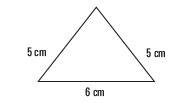12 cm2

3. Put these angles in order, draw them and classify them as acute, obtuse, or reflex:
212°, 62°, 87°, 134°, 1/4 turn, 7/8 of a turn, 23°.

23° 62° 87°, 1/4 turn, 134°, 212°, 7/8 of a turn (acute, acute, acute, right, obtuse, reflex, reflex)

4. A new hopscotch game is to be painted on a playground. On the plan the game is 2 cm wide and 7 cm long. The game on the playground will be simi­lar to the one on the plan. If the playground game is to be 60 cm wide, how long will it be?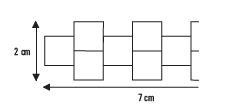410 cm or 2.1 m

5. Add two squares to each of these shapes to make them have exactly two lines of symmetry.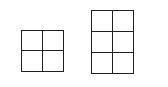One possible answer for each is shown below: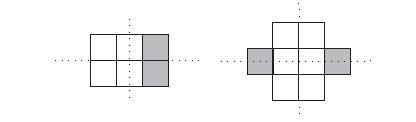6. Add two squares to each of the following shapes to make them have rota­tional symmetry of order 4.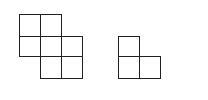One possible answer for each is shown below: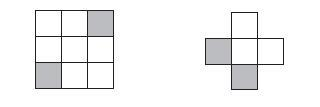7. (a) Hexominoes are formed when six identical squares are joined contig­uously, i.e. they are touching along at least one side, not just corner to corner. How many different, i.e not congruent, hexominoes are there? (Clue: there are more than 11 as we already know that 11 of them form nets for the cube.)

(b) Applying consistent naming for polyhedra, what would be an alternative name for the cube?

(a) There are 35 hexominoes. (b) A regular hexahedron.

(a) Name four different types of triangle.

(b) Name four different types of quadrilateral.

(c) Name four transformations that can be applied to 2-D shapes.

(a) Types of triangle – equilateral, isosceles, scalene, right-angled (scalene or isosceles)

(b) Types of quadrilateral – (any four from) square, rectangle, parallelogram, rhombus, kite, trapezium, isosceles trapezium

(c) The four transformations that can be applied to 2-D shapes – translation, reflection, rotation and enlargement

9. In describing 3-D shapes, define the following terms:

(a) Face
(b) Edge
(c) Vertex

(a) Face – the flat surface of a solid shape

(b) Edge – the line where two faces join

(c) Vertex – the point of intersection of edges

10. In which quadrants would the following Cartesian co-ordinates be?

(a) (2, -6)
(b) (-4, 7)
(c) (2, 4)
(d) (-3, -4)